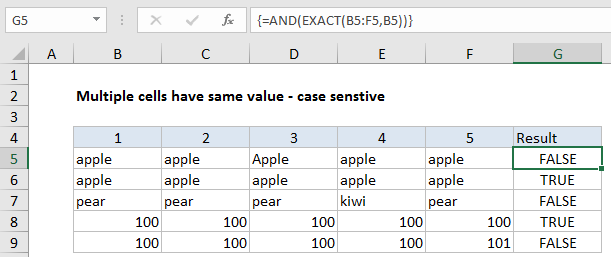## Excel Office

Excel How Tos, Tutorials, Tips & Tricks, Shortcuts

# Check if multiple cells have same value with case sensitive in Excel

To verify that multiple cells have the same value with a case-sensitive formula, you can use a simple array formula based on the EXACT and AND functions. See example below:

## Formula

`{=AND(EXACT(range,value))}`## Explanation

In the example shown, the formula in G5 is:

`=AND(EXACT(B5:F5,B5))`

This is an array formula and must be entered with control + shift + enter

Worked Example:   Cash denomination calculator in Excel

### How this formula works

This formula uses the EXACT formula to compare a range of cells to a single value:

`=EXACT(B5:F5,B5)`

Because we give EXACT a range of values in the first argument, we get back an array result containing TRUE FALSE values:

`{TRUE,FALSE,TRUE,TRUE,TRUE}`

This array goes into the AND function, which returns TRUE only if all values in the array are TRUE.

Worked Example:   Count dates in given year in Excel

### Ignore empty cells

To ignore empty cells, but still treat non-empty cells in a case-sensitive manner, you can use a version of the formula based on SUMPRODUCT:

`=SUMPRODUCT(--(EXACT(range,value)))=COUNTA(range)`

Here, we count exact matches using the same EXACT formula above, get a total count with SUMPRODUCT, and compare the result to a count of all non-empty cells, determined by COUNTA.

Worked Example:   How to get address of last cell in range in Excel

This is an array formula but control + shift + enter is not required because SUMPRODUCT handles the array natively.